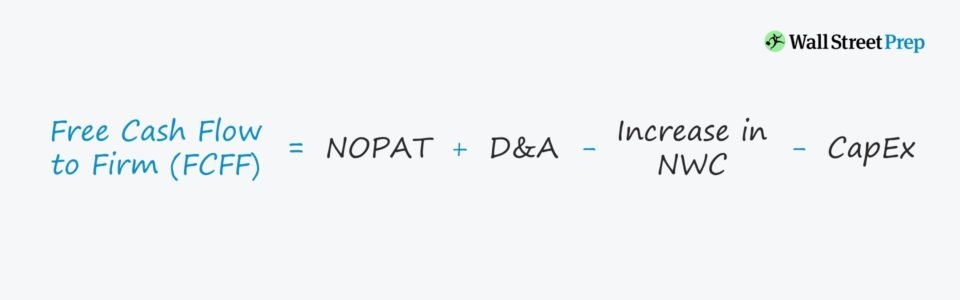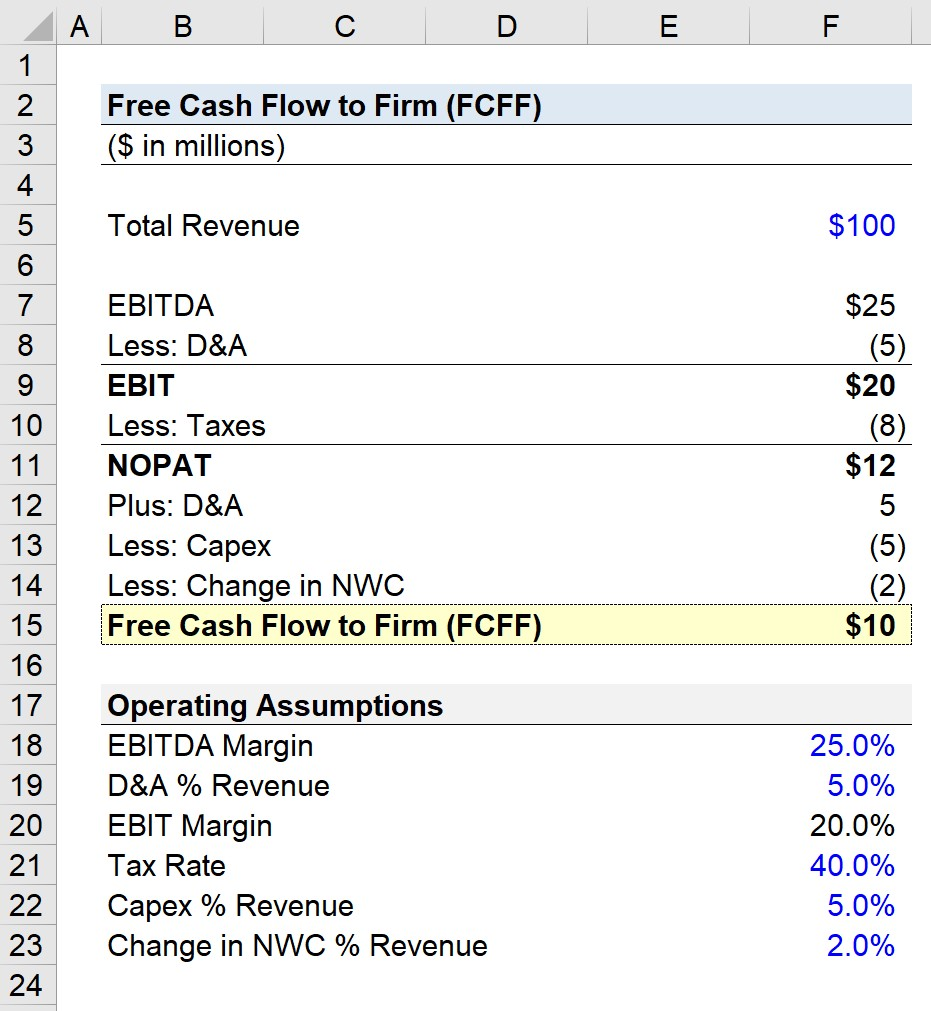# Free Cash Flow to Firm (FCFF)

Guide to Understanding Free Cash Flow to Firm (FCFF)## How to Calculate FCFF (Step-by-Step)

The free cash flow to firm (FCFF) metric is the cash available to all the firm’s creditors and common/preferred shareholders as generated from the core operations of the business and after accounting for expenses and long-term investments necessary to remain operating.

Before we discuss the formulas used to calculate the free cash flow to firm (FCFF), it is important to cover what this metric is intended to portray and discuss the standards used to determine which types of items should be included (and excluded).

• Core Operations: The FCFF value must reflect only the core operations of the business – each line item included should be strictly from the recurring sale of goods/services provided. For example, the cash proceeds from a one-time asset sale should be left out of the calculation as it is neither recurring nor is it part of the nature of the business.
• Normalization: The FCFF figures should also be normalized to set apart the recurring performance of the company. Given that one of the main use-cases of FCFF is for projection models, most notably the discounted cash flow (DCF), each item must be expected to be ongoing into the future.
• Discretionary Items: The discretionary line items that pertain to only one specific group (e.g., dividends) should also be excluded. This ties back to the theme of FCFF being applicable to all providers of capital. The payout of dividends benefits only equity shareholders and is a discretionary decision up to management while being unrelated to the core operations.
• Stakeholders Representations: FCFF corresponds with the enterprise value (TEV) and the weighted average cost of capital (WACC) as the three metrics all represent all stakeholders in a company.

## FCFF Formula

To calculate FCFF starting from earnings before interest and taxes (EBIT), we begin by adjusting EBIT for taxes.

EBIT is an unlevered profit measure since it is above the interest expense line and does not include outflows specific to one capital provider group (e.g., lenders).

The tax-effected EBIT is also commonly known as:

• EBIAT: “Earnings Before Interest After Taxes”
• NOPAT: “Net Operating Profit After Taxes”
NOPAT = EBIT * (1 Tax Rate %)

Next, non-cash items such as depreciation & amortization (D&A) are added back since they are not real cash outflows.

However, recall each item must be recurring and part of the core operations – thereby, not all non-cash items are added back (e.g., inventory write-downs).

Then, capital expenditures (capex) and the change in net working capital (NWC) are deducted.

Of the outflows in the cash from investing section, the line item that should be accounted for is capex.

The reasoning for this is that capex is required for operations to sustain into the future, especially maintenance capex.

The relationship between the change in NWC and free cash flow is as follows:

• Increase in NWC → Less FCF
• Decrease in NWC → More FCF

To provide two examples explaining the rationale behind NWC:

• Increase in Current Operating Asset: If a current operating asset such as accounts receivable (A/R) were to increase, that means the company is being less efficient at collecting cash from customers that paid on credit – in effect, the amount of cash on hand is reduced
• Increase in Current Operating Liability: If a current operating liability like accounts payable (A/P) were to increase, then that suggests the company has not yet paid suppliers/vendors for due payments – while the payment will still eventually be paid out, for the time being, the cash is in the possession of the company

Capex and increases in NWC each represent outflows of cash, which means less free cash flow remains post-operations for payments related to servicing interest, debt amortization, etc.

Putting this all together, the FCFF formula is as follows.

Free Cash Flow to Firm (FCFF) = NOPAT + D&A Change in NWC Capex

## Normalization Adjustments to Free Cash Flow to Firm

Normalizing the cash flows becomes particularly relevant when performing trading comps using FCFF-based multiples, in which the target company and its comparables (i.e., peer group) are benchmarked against each other.

For the comparison to be as close to being “apples to apples” as possible, the non-core operating income/(expenses) and non-recurring items should be adjusted out to prevent the output from being skewed.

## FCFF Calculator – Excel Template

We’ll now move to a modeling exercise, which you can access by filling out the form below.Submitting ...

## Step 1. FCFF Calculation Example (Calculate FCFF from EBITDA)

If we start the calculation from EBITDA, the minor difference is that D&A is subtracted and then added back later – and so, the net impact is the tax savings from the D&A.

Based on the assumptions listed, the EBITDA is \$25m, from which we deduct \$5m in D&A to get \$20m as the EBIT. And to calculate NOPAT, we apply a 40% tax rate to the \$20m of EBIT, which comes out to \$12m.

From the \$12m in NOPAT, we add back the \$5m in D&A and then finish the calculation by subtracting the \$5m in capex and \$2m in the change in NWC – for an FCFF of \$10m.## Step 2. FCFF Calculation Example (Net Income to FCFF)

An alternative formula to calculate FCFF starts with net income, which is a post-tax and interest metric.

FCFF = Net Income + D&A + [Interest Expense * (1 Tax Rate)] Change in NWC Capex

Next, we add back the relevant non-cash expenses, like D&A.

The D&A and change in NWC adjustments to net income could be thought of as being analogous to calculating the cash flow from operations (CFO) section of the cash flow statement. Then, the interest expense is added back, since it pertains only to lenders.

In addition, the “tax shield” associated with interest must be added back too (i.e., the tax savings). The interest on debt lowered the taxable income – thus, the interest must be multiplied by (1 – Tax Rate).

In effect, the impact of interest is removed from the taxes – which is the objective of NOPAT (i.e., capital-structure neutral).

To make sure this point is clear, the FCFF is available to both creditors and equity holders, so we are working towards calculating figures on a “before interest” basis since we are starting from CFO (i.e., an after-tax metric).

Therefore, to get to a value that represents all providers of capital, we add back the interest expense amount as adjusted for the fact that interest is tax-deductible.

Now that net income had D&A added to it and is now free of debt-related payments (and side impacts), we can proceed with deducting the re-investment needs: the change in NWC and Capex.

## Step 3. FCFF Calculation Example (Cash from Operations to FCFF)

The next formula for calculating FCFF starts off with cash flow from operations (CFO).

FCFF = CFO + [Interest Expense * (1 Tax Rate)] Capex

On the cash flow statement, the CFO section has the “bottom line” from the income statement at the top, which is then adjusted for non-cash expenses and changes in working capital.

However, be careful not to just pull the CFO figure from the financial statements without confirming the non-cash charges are indeed related to the core operations and are recurring.

After doing so, we add back the tax-adjusted interest expense, following the same logic as the prior formula.

In the final step, we subtract capex, since it represents a required cash outlay.

There is no need to deduct the change in NWC this time around since CFO already takes it into account.

But capex is located in the cash flow from investing section, and thus was not yet accounted for.Step-by-Step Online Course

### Everything You Need To Master Financial Modeling

Enroll in The Premium Package: Learn Financial Statement Modeling, DCF, M&A, LBO and Comps. The same training program used at top investment banks.Inline FeedbacksLearn Financial Modeling Online

Everything you need to master financial and valuation modeling: 3-Statement Modeling, DCF, Comps, M&A and LBO.

X

The Wall Street Prep Quicklesson Series

7 Free Financial Modeling Lessons

Get instant access to video lessons taught by experienced investment bankers. Learn financial statement modeling, DCF, M&A, LBO, Comps and Excel shortcuts.